### IMO Shortlist 2018 problem G2

Kvaliteta:
Avg: 0,0
Težina:
Avg: 6,0

Let$ABC$ be a triangle with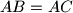$AB=AC$, and let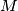$M$ be the midpoint of$BC$. Let$P$ be a point such that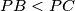$PB and$PA$ is parallel to$BC$. Let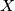$X$ and$Y$ be points on the lines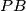$PB$ and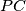$PC$, respectively, so that$B$ lies on the segment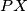$PX$,$C$ lies on the segment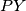$PY$, and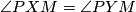$\angle PXM=\angle PYM$. Prove that the quadrilateral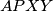$APXY$ is cyclic.

Izvor: https://www.imo-official.org/problems/IMO2018SL.pdf